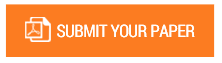Improve Performance of MPPT Photovoltaic System by PID Controller

Authors : Mahendra Kumar Meena ,Deepesh Namdev .

Volume/Issue : Volume 1 - 2016, Issue 4 - July

Maximum power point trackers are efficient enough to enhance an efficiency of the photovoltaic system. So many methodologies have been proposed up to this date to achieve maximum power. PV modules are capable of generating maximum power under diverse atmospheric conditions. The base paper  proposed an MPPT (Maximum Power Point Tracking) algorithm that based on fuzzy logic for a solar system. The solar panel is analyzed and then simulated by using SIMULINK or MATLAB. The PV system is bound to DC-DC BUCK BOOST converter. The MPP (Maximum Power Point) is an operating point where Solar panel used to generate maximum power. In order to gain maximum efficiency and the power, it is required that entire system should operate at MPP. The MPP of PV panel maintains similar on changing by changing cell temperature and solar irradiance. So to achieve maximum power with PV system, the MPPT algorithms are put into the operation. In this paper, we are enhancing voltage, current and power performance of solar output. Hence, further, the application of PID controller helps to enhance performance that we are going to implement. PID controller is efficient enough to enhance solar output power performance.

Keywords : PV Modeling, PID controller, Buck-Boost Converter, Fuzzy Logic, MPPT.

#### CALL FOR PAPERS

Paper Submission Last Date
31 - May - 2022